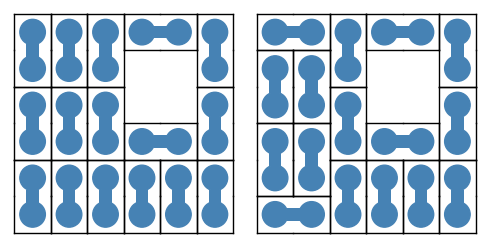# Revision history [back]

Sage has a built-in class of polyominos, with some solving and plotting functions.

Here is an example.

Some preliminary imports.

sage: import itertools
sage: from sage.combinat.tiling import TilingSolver, Polyomino


Define a polyomino and a chessboard.

sage: domino = Polyomino([(0, 0), (1, 0)], color='steelblue')
sage: s = set(itertools.product(range(6), repeat=2))
sage: s.difference_update([(3, 3), (3, 4), (4, 3), (4, 4)])
sage: chessboard = Polyomino(s)


Define the tiling problem.

sage: T = TilingSolver([domino], box=chessboard, reflection=False, rotation=True, reusable=True)


Count solutions.

sage: T.number_of_solutions()


Store the solutions in a list.

sage: solutions = list(T.solve())


Turn two of the solutions into graphic objects.

sage: G = sum([piece.show2d() for piece in solutions], Graphics())
sage: GG = sum([piece.show2d() for piece in solutions], Graphics())


Save them to file, or view them.

sage: graphics_array([G, GG]).save("domino-tilings.png", aspect_ratio=1, axes=False)
sage: graphics_array([G, GG]).show(aspect_ratio=1, axes=False, figsize=5)


Sage has a built-in class of polyominos, with some solving and plotting functions.

Here is an example.

Some preliminary imports.

sage: import itertools
sage: from sage.combinat.tiling import TilingSolver, Polyomino


Define a polyomino and a chessboard.

sage: domino = Polyomino([(0, 0), (1, 0)], color='steelblue')
sage: s = set(itertools.product(range(6), repeat=2))
sage: s.difference_update([(3, 3), (3, 4), (4, 3), (4, 4)])
sage: chessboard = Polyomino(s)


Define the tiling problem.

sage: T = TilingSolver([domino], box=chessboard, reflection=False, rotation=True, reusable=True)


Count solutions.

sage: T.number_of_solutions()


Store the solutions in a list.

sage: solutions = list(T.solve())


Turn two of the solutions into graphic objects.

sage: G = sum([piece.show2d() for piece in solutions], Graphics())
sage: GG = sum([piece.show2d() for piece in solutions], Graphics())


Save them to file, or view them.

sage: graphics_array([G, GG]).save("domino-tilings.png", aspect_ratio=1, axes=False)
sage: graphics_array([G, GG]).show(aspect_ratio=1, axes=False, figsize=5)